# NMath User's Guide

21.5 Iteratively Reweighted Least Squares (.NET, C#, CSharp, VB, Visual Basic, F#)

Iteratively reweighted least squares (IRLS) is an iterative minimization technique in which each step involves solving a standard weighted least squares (Section 21.4). New weights are computed at each iteration by applying a weighting function to the current residuals. The process terminates when either a specified convergence function returns true—typically when either the residuals or the solution are unchanged on successive iterations—or when a specified maximum number of iterations is reached.

NMath provides class for solving IRLS problems. The default weighting function is a bisquare weighting function, and the default convergence function returns true when the solutions do not change, within tolerance, on successive iterations. For instance:

Code Example – C# iteratively reweighted least squares

```var irls = new DoubleIterativelyReweightedLeastSq();
```

Code Example – VB iteratively reweighted least squares

```Dim IRLS As New DoubleIterativelyReweightedLeastSq()
```

Additional constructors enable you to specify the tolerance, the maximum number of iterations, and a weighting function (see below). Properties Tolerance, MaxIterations, and WeightsFunction are also provided for modifying these values post-construction.

The Solve() method solves the least squares problem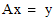for x using the IRLS method:

Code Example – C# iteratively reweighted least squares

```DoubleVector x = irls.Solve( A, y );
```

Code Example – VB iteratively reweighted least squares

```Dim X As DoubleVector = IRLS.Solve(A, Y)
```

Property Residuals gets the residual vector from the most recent computation. Iterations gets the number of iterations performed. For instance:

Code Example – C# iteratively reweighted least squares

```if ( irls.MaxIterationsMet ) {
```
```  Console.WriteLine(
```
```    "The algorithm failed to converge in {0} iterations.",
```
```    irls.MaxIterations
```
```  );
```
```}
```
```else {
```
```  Console.WriteLine(
```
```    "Algorithm converged in {0} iterations.", irls.Iterations );
```
```}
```

Code Example – VB iteratively reweighted least squares

```If IRLS.MaxIterationsMet Then
```
```  Console.WriteLine(
```
```    "The algorithm failed to converge in {0} iterations.",
```
```    IRLS.MaxIterations)
```
```Else
```
```  Console.WriteLine(
```
```    "Algorithm converged in {0} iterations.", IRLS.Iterations)
```
```End If
```

Convergence Functions

The convergence function is called at the end of each iteration. If the function returns true, the algorithm is terminated; otherwise, iteration continues.

Convergence functions are specified as instances of delegate DoubleIterativelyReweightedLeastSq.ToleranceMetFunction:

Code Example – C# iteratively reweighted least squares

```public delegate bool ToleranceMetFunction(
```
```  double tolerance,
```
```  DoubleVector lastSolution,
```
```  DoubleVector currentSolution,
```
```  DoubleVector lastResiduals,
```
```  DoubleVector currentResiduals);
```

Code Example – VB iteratively reweighted least squares

```Delegate Function ToleranceMetFunction(
```
```  Tolerance As Double,
```
```  LastSolution As DoubleVector,
```
```  CurrentSolution As DoubleVector,
```
```  LastResiduals As DoubleVector,
```
```  CurrentResiduals As DoubleVector) As Boolean
```

For example, this code encapsulates the user-defined function MyFunction as a DoubleIterativelyReweightedLeastSq.ToleranceMetFunction delegate:

Code Example – C# iteratively reweighted least squares

```public static bool MyFunction(
```
```  double tolerance,
```
```  DoubleVector lastSolution,
```
```  DoubleVector currentSolution,
```
```  DoubleVector lastResiduals,
```
```  DoubleVector currentResiduals )
```
```{
```
```  double a =
```
```    NMathFunctions.MaxAbsValue( currentResiduals - lastResiduals );
```
```  double b = NMathFunctions.MaxAbsValue( currentResiduals );
```
```  return ( a/b ) < tolerance;
```
```}

```

```public static
```
``` DoubleIterativelyReweightedLeastSq.ToleranceMetFunction
```
```   residualsUnchanged =
```
```    new DoubleIterativelyReweightedLeastSq.ToleranceMetFunction(
```
```      MyFunction );
```

Code Example – VB iteratively reweighted least squares

```public Shared Function MyFunction( Tolerance As Double, ,
```
```  LastSolution As DoubleVector, CurrentSolution As DoubleVector,
```
```  LastResiduals As DoubleVector, CurrentResiduals As DoubleVector)
```
```As Boolean
```
```  Dim A As Double =
```
```    NMathFunctions.MaxAbsValue(CurrentResiduals - LastResiduals)
```
```  Dim B As Double = NMathFunctions.MaxAbsValue(CurrentResiduals)
```
```  Return (A / B) < Tolerance
```
```End Function
```

Property ConvergenceFunction can be used to get and set the convergence function on a DoubleIterativelyReweightedLeastSq instance:

Code Example – C# iteratively reweighted least squares

```irls.ConvergenceFunction = residualsUnchanged;
```

Code Example – VB iteratively reweighted least squares

```IRLS.ConvergenceFunction = ResidualsUnchanged
```

Weighting Functions

NMath provides a selection of least squares weighting functions. Typical weighting functions used in IRLS are a function of the adjusted residuals from the previous iteration. Abstract base class provides method AdjustedResiduals() for calculating the adjusted residuals according to the following formula: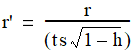where

r is the actual residuals.

t is a tuning constant by which the residuals are divided before computing weights. Decreasing the tuning constant increases the downweight assigned to large residuals, and increasing the tuning constant decreases the downweight assigned to large residuals.

h is the vector of leverage values that adjust the residuals by downweighting high-leverage data points, which have a large effect on the least squares fit. The leverage values are the main diagonal of the hat matrix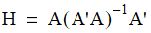s is an estimate of the standard deviation of the error term given bywhere MAD is the median absolute deviation of the residuals from their median. The constant 0.6745 makes the estimate unbiased for the normal distribution.

DoubleLeastSqWeightingFunction also implements the interface, which provides methods Initialize() for performing any needed initialization given the matrix A, and GetWeights() for computing weights from a given vector of residuals.

Two concrete implementations of DoubleLeastSqWeightingFunction are provided:

applies the bisquare weighting formula to a set of adjusted residuals: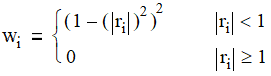where r is the adjusted residuals computed by the AdjustedResidual() function on the base class DoubleLeastSqWeightingFunction.

applies the fair weighting formula to a set of adjusted residuals: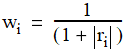where r is the adjusted residuals computed by the AdjustedResidual() function on the base class DoubleLeastSqWeightingFunction.

The default weighting function used is the bisquare weighting function. This code constructs a DoubleIterativelyReweightedLeastSq instance using the fair weighting function:

Code Example – C# iteratively reweighted least squares

```var weightingFunction = DoubleFairWeightingFunction();
```
```var irls = new DoubleIterativelyReweightedLeastSq(
weightingFunction );

```

Code Example – VB iteratively reweighted least squares

```Dim WeightingFunction As IDoubleLeastSqWeightingFunction =
```
```  New DoubleFairWeightingFunction()
```
```Dim IRLS As New
```
```  DoubleIterativelyReweightedLeastSq(WeightingFunction)
```

The weighting function can also be changed on an existing DoubleIterativelyReweightedLeastSq object using the WeightsFunction property:

Code Example – C# iteratively reweighted least squares

```irls.WeightsFunction = new DoubleFairWeightingFunction();
```

Code Example – C# iteratively reweighted least squares

```IRLS.WeightsFunction = New DoubleFairWeightingFunction()

```

Top

Top